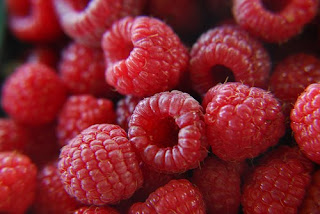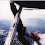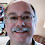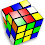## Wednesday, July 04, 2012

### GeekDad Puzzle of the Week: Poaching BerriesGeekDad Puzzle of the Week: Poaching Berries:
Q: Leif and Kestrel are willing to give GeekDad a 20% cut of berries they poach for turning a blind eye. Imagine that Leif picks five berries per poach and Kestrel picks three berries per poach and that they attempt to poach once every day, with the exception of any day just after they’ve been caught. Now imagine that each time they poach berries, they have a 15% chance of getting caught. How many berries can GeekDad expect to eat each each week, averaged over time?
I'll post my thoughts on the answer after the answer is revealed (generally early next week).

Edit: The following answer was what I submitted to end up winning the puzzle:

Let's assume a few things:
1) Leif and Kestrel start in a state where they haven't ever been caught.
2) Each time they are caught, the berries that day don't count. Also, they must skip the next day until trying again.

There are essentially 3 states that each child could be in. Since the probabilities for each child are the same we can group them together, though in reality the puzzle allows for one child to be caught and the other not. The results work out the same, so let's just simplify it to a pair of children trying to poach 8 berries a day.

The children could be:
1) Caught the day before and therefore have no chance of getting berries that day (must sit out).
2) Caught today (15%, if not caught the day before)
3) Not caught today (85%, if not caught the day before)

Each day, the chance of being caught the day before is just carried forward. So on day 2, the chance is 15% they are sitting out. That leaves 85% chance they are attempting poaching. Of that 85%, there's a 15% chance they are caught (0.85 x 0.15 = 12.75%) and an 85% chance they poach successfully (0.85 x 0.85 = 72.25%).

If you repeat this, the next day (Day 3) they have a 12.75% chance of sitting out and a 87.25% chance of attempting poaching. Day 4, the chance of sitting out is 0.15 x 87.25% = 13.0875% and not sitting out is 86.9125%. Day 5, the chance of sitting out is 0.15 x 86.9125% = 13.036875% and not sitting out is 86.963125%. You can continue this progression and you will see that the chance they are sitting out approaches a value of about 13.043478%. The chance they are caught that day is equivalently about 13.043478%. That leaves a 73.913043% chance they are able to poach 8 berries with an expected return of 5.913043478 berries a day.

That equates to approximately 41.39130435 berries a week. With a 20% "commission" after awhile you will be eating approximately 8.27826087 berries each week.

Note: For a more accurate answer (rather than just a decimal approximation) we can solve this algebraically as follows:

Let p be the chance that you ARE sitting out.
Let q be the chance you are NOT sitting out.

Together these are mutually exclusive and therefore add up to 100% (or mathematically we say 1)
p + q = 1
p = 1 - q

The chance you are NOT sitting out, but CAUGHT is 0.15q
The chance you are NOT sitting out, and SUCCESSFULLY POACHED is 0.85q

We know that eventually the two values p and 0.15q end up being the same, so equate them
p = 0.15q

Substitute in 1-q:
1 - q = 0.15q

Rearrange:
1 = 1.15q
q = 1/1.15

The chance we are caught that day is 0.15q
0.15q = 0.15(1/1.15) = 0.15/1.15 = 15/115 = 3/23
And the chance we poach some berries is 0.85q
0.85q = 0.85(1/1.15) = 0.85/1.15 = 85/115 = 17/23

For the sake of completeness that means you have:
3/23 = chance child is sitting out
3/23 = chance child was caught today
17/23 = chance child was able to poach successfully

Now multiply this last number by 8 berries attempted times 7 days and then times 1/5 (20% commission) to get the expected number of berries poached each week.
Berries per week = 17/23 x 8 x 7 x 1/5

If you reduce that to a fraction you end up with:
952/115 = 8 32/115 berries each week.

A: 8 32/115 berries each week.
8.27826086956521739130434... (underlined portion repeats indefinitely)

1.I'm not sure, but he most likely will need Lomotil.

2.# of berries x 7 x 0.15.

3.Didn't Khrushchev say he would berry us?

4.This comment has been removed by the author.

5.Sorry, I hadn't realized that this was an actual contest, as opposed to a recreational discussion. So, I've deleted my analysis of the problem, which I'll re-post once the answer is out.

1.But feel free to email your analysis to the GeekDad email and join in on the chance to win a \$50 gift certificate.

6.In retrospect, the 3 states (sitting out, caught, poaching) happen with the following ratios:
15:15:85

So that's 15/115, 15/115, 85/115
Reducing we have 3/23, 3/23, 17/23

If the probability of poaching is 17/23, then the expected return is 17/23 x 8 berries. And a 20% commission on a weeks worth of berries is:
17/23 x 8 x 7 x 1/5
= 8 32/115 berries.